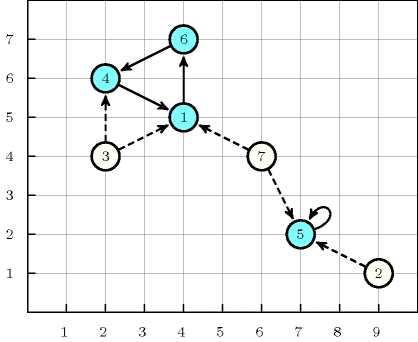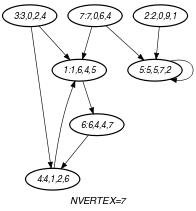## 5.105. cycle_or_accessibility

Origin

Inspired by [LabbeLaporteRodriguezMartin98].

Constraint

$\mathrm{𝚌𝚢𝚌𝚕𝚎}_\mathrm{𝚘𝚛}_\mathrm{𝚊𝚌𝚌𝚎𝚜𝚜𝚒𝚋𝚒𝚕𝚒𝚝𝚢}\left(\mathrm{𝙼𝙰𝚇𝙳𝙸𝚂𝚃},\mathrm{𝙽𝙲𝚈𝙲𝙻𝙴},\mathrm{𝙽𝙾𝙳𝙴𝚂}\right)$

Arguments
 $\mathrm{𝙼𝙰𝚇𝙳𝙸𝚂𝚃}$ $\mathrm{𝚒𝚗𝚝}$ $\mathrm{𝙽𝙲𝚈𝙲𝙻𝙴}$ $\mathrm{𝚍𝚟𝚊𝚛}$ $\mathrm{𝙽𝙾𝙳𝙴𝚂}$ $\mathrm{𝚌𝚘𝚕𝚕𝚎𝚌𝚝𝚒𝚘𝚗}\left(\mathrm{𝚒𝚗𝚍𝚎𝚡}-\mathrm{𝚒𝚗𝚝},\mathrm{𝚜𝚞𝚌𝚌}-\mathrm{𝚍𝚟𝚊𝚛},𝚡-\mathrm{𝚒𝚗𝚝},𝚢-\mathrm{𝚒𝚗𝚝}\right)$
Restrictions
 $\mathrm{𝙼𝙰𝚇𝙳𝙸𝚂𝚃}\ge 0$ $\mathrm{𝙽𝙲𝚈𝙲𝙻𝙴}\ge 1$ $\mathrm{𝙽𝙲𝚈𝙲𝙻𝙴}\le |\mathrm{𝙽𝙾𝙳𝙴𝚂}|$ $\mathrm{𝚛𝚎𝚚𝚞𝚒𝚛𝚎𝚍}$$\left(\mathrm{𝙽𝙾𝙳𝙴𝚂},\left[\mathrm{𝚒𝚗𝚍𝚎𝚡},\mathrm{𝚜𝚞𝚌𝚌},𝚡,𝚢\right]\right)$ $\mathrm{𝙽𝙾𝙳𝙴𝚂}.\mathrm{𝚒𝚗𝚍𝚎𝚡}\ge 1$ $\mathrm{𝙽𝙾𝙳𝙴𝚂}.\mathrm{𝚒𝚗𝚍𝚎𝚡}\le |\mathrm{𝙽𝙾𝙳𝙴𝚂}|$ $\mathrm{𝚍𝚒𝚜𝚝𝚒𝚗𝚌𝚝}$$\left(\mathrm{𝙽𝙾𝙳𝙴𝚂},\mathrm{𝚒𝚗𝚍𝚎𝚡}\right)$ $\mathrm{𝙽𝙾𝙳𝙴𝚂}.\mathrm{𝚜𝚞𝚌𝚌}\ge 0$ $\mathrm{𝙽𝙾𝙳𝙴𝚂}.\mathrm{𝚜𝚞𝚌𝚌}\le |\mathrm{𝙽𝙾𝙳𝙴𝚂}|$ $\mathrm{𝙽𝙾𝙳𝙴𝚂}.𝚡\ge 0$ $\mathrm{𝙽𝙾𝙳𝙴𝚂}.𝚢\ge 0$
Purpose

Consider a digraph $G$ described by the $\mathrm{𝙽𝙾𝙳𝙴𝚂}$ collection. Cover a subset of the vertices of $G$ by a set of vertex-disjoint circuits in such a way that the following property holds: for each uncovered vertex ${v}_{1}$ of $G$ there exists at least one covered vertex ${v}_{2}$ of $G$ such that the Manhattan distance between ${v}_{1}$ and ${v}_{2}$ is less than or equal to $\mathrm{𝙼𝙰𝚇𝙳𝙸𝚂𝚃}$.

Example
$\left(\begin{array}{c}3,2,〈\begin{array}{cccc}\mathrm{𝚒𝚗𝚍𝚎𝚡}-1\hfill & \mathrm{𝚜𝚞𝚌𝚌}-6\hfill & 𝚡-4\hfill & 𝚢-5,\hfill \\ \mathrm{𝚒𝚗𝚍𝚎𝚡}-2\hfill & \mathrm{𝚜𝚞𝚌𝚌}-0\hfill & 𝚡-9\hfill & 𝚢-1,\hfill \\ \mathrm{𝚒𝚗𝚍𝚎𝚡}-3\hfill & \mathrm{𝚜𝚞𝚌𝚌}-0\hfill & 𝚡-2\hfill & 𝚢-4,\hfill \\ \mathrm{𝚒𝚗𝚍𝚎𝚡}-4\hfill & \mathrm{𝚜𝚞𝚌𝚌}-1\hfill & 𝚡-2\hfill & 𝚢-6,\hfill \\ \mathrm{𝚒𝚗𝚍𝚎𝚡}-5\hfill & \mathrm{𝚜𝚞𝚌𝚌}-5\hfill & 𝚡-7\hfill & 𝚢-2,\hfill \\ \mathrm{𝚒𝚗𝚍𝚎𝚡}-6\hfill & \mathrm{𝚜𝚞𝚌𝚌}-4\hfill & 𝚡-4\hfill & 𝚢-7,\hfill \\ \mathrm{𝚒𝚗𝚍𝚎𝚡}-7\hfill & \mathrm{𝚜𝚞𝚌𝚌}-0\hfill & 𝚡-6\hfill & 𝚢-4\hfill \end{array}〉\hfill \end{array}\right)$

Figure 5.105.1 represents the solution associated with the example. The covered vertices are coloured in blue, while the links starting from the uncovered vertices are dashed. The $\mathrm{𝚌𝚢𝚌𝚕𝚎}_\mathrm{𝚘𝚛}_\mathrm{𝚊𝚌𝚌𝚎𝚜𝚜𝚒𝚋𝚒𝚕𝚒𝚝𝚢}$ constraint holds since:

• In the solution we have $\mathrm{𝙽𝙲𝚈𝙲𝙻𝙴}=2$ disjoint circuits.

• All the 3 uncovered nodes are located at a distance that does not exceed $\mathrm{𝙼𝙰𝚇𝙳𝙸𝚂𝚃}=3$ from at least one covered node.

##### Figure 5.105.1. Final graph associated with the facilities location problemTypical
 $\mathrm{𝙼𝙰𝚇𝙳𝙸𝚂𝚃}>0$ $\mathrm{𝙽𝙲𝚈𝙲𝙻𝙴}<|\mathrm{𝙽𝙾𝙳𝙴𝚂}|$ $|\mathrm{𝙽𝙾𝙳𝙴𝚂}|>2$
Symmetries
• Items of $\mathrm{𝙽𝙾𝙳𝙴𝚂}$ are permutable.

• Attributes of $\mathrm{𝙽𝙾𝙳𝙴𝚂}$ are permutable w.r.t. permutation $\left(\mathrm{𝚒𝚗𝚍𝚎𝚡}\right)$ $\left(\mathrm{𝚜𝚞𝚌𝚌}\right)$ $\left(𝚡,𝚢\right)$ (permutation applied to all items).

• One and the same constant can be added to the $𝚡$ attribute of all items of $\mathrm{𝙽𝙾𝙳𝙴𝚂}$.

• One and the same constant can be added to the $𝚢$ attribute of all items of $\mathrm{𝙽𝙾𝙳𝙴𝚂}$.

Arg. properties

Functional dependency: $\mathrm{𝙽𝙲𝚈𝙲𝙻𝙴}$ determined by $\mathrm{𝙽𝙾𝙳𝙴𝚂}$.

Remark

This kind of facilities location problem is described in  [LabbeLaporteRodriguezMartin98] pages. In addition to our example they also mention the cost problem that is usually a trade-off between the vertices that are directly covered by circuits and the others.

Keywords
Arc input(s)

$\mathrm{𝙽𝙾𝙳𝙴𝚂}$

Arc generator
$\mathrm{𝐶𝐿𝐼𝑄𝑈𝐸}$$↦\mathrm{𝚌𝚘𝚕𝚕𝚎𝚌𝚝𝚒𝚘𝚗}\left(\mathrm{𝚗𝚘𝚍𝚎𝚜}\mathtt{1},\mathrm{𝚗𝚘𝚍𝚎𝚜}\mathtt{2}\right)$

Arc arity
Arc constraint(s)
$\mathrm{𝚗𝚘𝚍𝚎𝚜}\mathtt{1}.\mathrm{𝚜𝚞𝚌𝚌}=\mathrm{𝚗𝚘𝚍𝚎𝚜}\mathtt{2}.\mathrm{𝚒𝚗𝚍𝚎𝚡}$
Graph property(ies)
 $•$$\mathrm{𝐍𝐓𝐑𝐄𝐄}$$=0$ $•$$\mathrm{𝐍𝐂𝐂}$$=\mathrm{𝙽𝙲𝚈𝙲𝙻𝙴}$

Arc input(s)

$\mathrm{𝙽𝙾𝙳𝙴𝚂}$

Arc generator
$\mathrm{𝐶𝐿𝐼𝑄𝑈𝐸}$$↦\mathrm{𝚌𝚘𝚕𝚕𝚎𝚌𝚝𝚒𝚘𝚗}\left(\mathrm{𝚗𝚘𝚍𝚎𝚜}\mathtt{1},\mathrm{𝚗𝚘𝚍𝚎𝚜}\mathtt{2}\right)$

Arc arity
Arc constraint(s)
$\bigvee \left(\begin{array}{c}\mathrm{𝚗𝚘𝚍𝚎𝚜}\mathtt{1}.\mathrm{𝚜𝚞𝚌𝚌}=\mathrm{𝚗𝚘𝚍𝚎𝚜}\mathtt{2}.\mathrm{𝚒𝚗𝚍𝚎𝚡},\hfill \\ \bigwedge \left(\begin{array}{c}\mathrm{𝚗𝚘𝚍𝚎𝚜}\mathtt{1}.\mathrm{𝚜𝚞𝚌𝚌}=0,\hfill \\ \mathrm{𝚗𝚘𝚍𝚎𝚜}\mathtt{2}.\mathrm{𝚜𝚞𝚌𝚌}\ne 0,\hfill \\ \mathrm{𝚊𝚋𝚜}\left(\mathrm{𝚗𝚘𝚍𝚎𝚜}\mathtt{1}.𝚡-\mathrm{𝚗𝚘𝚍𝚎𝚜}\mathtt{2}.𝚡\right)+\mathrm{𝚊𝚋𝚜}\left(\mathrm{𝚗𝚘𝚍𝚎𝚜}\mathtt{1}.𝚢-\mathrm{𝚗𝚘𝚍𝚎𝚜}\mathtt{2}.𝚢\right)\le \mathrm{𝙼𝙰𝚇𝙳𝙸𝚂𝚃}\hfill \end{array}\right)\hfill \end{array}\right)$
Graph property(ies)
$\mathrm{𝐍𝐕𝐄𝐑𝐓𝐄𝐗}$$=|\mathrm{𝙽𝙾𝙳𝙴𝚂}|$

Sets
$\begin{array}{c}\mathrm{𝖯𝖱𝖤𝖣}↦\hfill \\ \left[\begin{array}{c}\mathrm{𝚟𝚊𝚛𝚒𝚊𝚋𝚕𝚎𝚜}-\mathrm{𝚌𝚘𝚕}\left(\begin{array}{c}\mathrm{𝚅𝙰𝚁𝙸𝙰𝙱𝙻𝙴𝚂}-\mathrm{𝚌𝚘𝚕𝚕𝚎𝚌𝚝𝚒𝚘𝚗}\left(\mathrm{𝚟𝚊𝚛}-\mathrm{𝚍𝚟𝚊𝚛}\right),\hfill \\ \mathrm{𝚒𝚝𝚎𝚖}\left(\mathrm{𝚟𝚊𝚛}-\mathrm{𝙽𝙾𝙳𝙴𝚂}.\mathrm{𝚜𝚞𝚌𝚌}\right)\right]\hfill \end{array}\right),\hfill \\ \mathrm{𝚍𝚎𝚜𝚝𝚒𝚗𝚊𝚝𝚒𝚘𝚗}\hfill \end{array}\right]\hfill \end{array}$

Constraint(s) on sets
$\mathrm{𝚗𝚟𝚊𝚕𝚞𝚎𝚜}_\mathrm{𝚎𝚡𝚌𝚎𝚙𝚝}_\mathtt{0}$$\left(\mathrm{𝚟𝚊𝚛𝚒𝚊𝚋𝚕𝚎𝚜},=,1\right)$
Graph model

For each vertex $v$ we have introduced the following attributes:

• $\mathrm{𝚒𝚗𝚍𝚎𝚡}$: the label associated with $v$,

• $\mathrm{𝚜𝚞𝚌𝚌}$: if $v$ is not covered by a circuit then 0; If $v$ is covered by a circuit then index of the successor of $v$.

• $𝚡$: the $𝚡$-coordinate of $v$,

• $𝚢$: the $𝚢$-coordinate of $v$.

The first graph constraint forces all vertices, which have a non-zero successor, to form a set of $\mathrm{𝙽𝙲𝚈𝙲𝙻𝙴}$ vertex-disjoint circuits.

The final graph associated with the second graph constraint contains two types of arcs:

• The arcs belonging to one circuit (i.e., $\mathrm{𝚗𝚘𝚍𝚎𝚜}\mathtt{1}.\mathrm{𝚜𝚞𝚌𝚌}=\mathrm{𝚗𝚘𝚍𝚎𝚜}\mathtt{2}.\mathrm{𝚒𝚗𝚍𝚎𝚡}$),

• The arcs between one vertex ${v}_{1}$ that does not belong to any circuit (i.e., $\mathrm{𝚗𝚘𝚍𝚎𝚜}\mathtt{1}.\mathrm{𝚜𝚞𝚌𝚌}=0$) and one vertex ${v}_{2}$ located on a circuit (i.e., $\mathrm{𝚗𝚘𝚍𝚎𝚜}\mathtt{2}.\mathrm{𝚜𝚞𝚌𝚌}\ne 0$) such that the Manhattan distance between ${v}_{1}$ and ${v}_{2}$ is less than or equal to $\mathrm{𝙼𝙰𝚇𝙳𝙸𝚂𝚃}$.

In order to specify the fact that each vertex is involved in at least one arc we use the graph property $\mathrm{𝐍𝐕𝐄𝐑𝐓𝐄𝐗}$ $=$ $|\mathrm{𝙽𝙾𝙳𝙴𝚂}|$. Finally the dynamic constraint $\mathrm{𝚗𝚟𝚊𝚕𝚞𝚎𝚜}_\mathrm{𝚎𝚡𝚌𝚎𝚙𝚝}_\mathtt{0}\left(\mathrm{𝚟𝚊𝚛𝚒𝚊𝚋𝚕𝚎𝚜},=,1\right)$ expresses the fact that, for each vertex $v$, there is exactly one predecessor of $v$ that belongs to a circuit.

Parts (A) and (B) of Figure 5.105.2 respectively show the initial and final graph associated with the second graph constraint of the Example slot.

##### Figure 5.105.2. Initial and final graph of the $\mathrm{𝚌𝚢𝚌𝚕𝚎}_\mathrm{𝚘𝚛}_\mathrm{𝚊𝚌𝚌𝚎𝚜𝚜𝚒𝚋𝚒𝚕𝚒𝚝𝚢}$ constraint(a) (b)
Signature

Since $|\mathrm{𝙽𝙾𝙳𝙴𝚂}|$ is the maximum number of vertices of the final graph associated with the second graph constraint we can rewrite $\mathrm{𝐍𝐕𝐄𝐑𝐓𝐄𝐗}$ $=$ $|\mathrm{𝙽𝙾𝙳𝙴𝚂}|$ to $\mathrm{𝐍𝐕𝐄𝐑𝐓𝐄𝐗}$ $\ge$ $|\mathrm{𝙽𝙾𝙳𝙴𝚂}|$. This leads to simplify $\underline{\overline{\mathrm{𝐍𝐕𝐄𝐑𝐓𝐄𝐗}}}$ to $\overline{\mathrm{𝐍𝐕𝐄𝐑𝐓𝐄𝐗}}$.